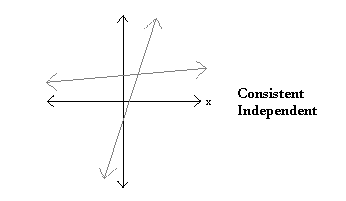# Parallel & Perpendicular Lines

## What does it mean?

### Definitions:

If the two equations describe lines that intersect once, the system is independent and consistent.If the two equations describe parallel lines, and thus lines that do not intersect, the system is independent and inconsistent.If the two equations describe the same line, and thus lines that intersect an infinite number of times, the system is dependent and consistent.The following chart will help determine if an equation is consistent and if an equation is dependent:## Video

### Equations of Parallel and Perpendicular Lines

Watch a Khan Academy Video »
Length: 9:13

## Video

### Perpendicular Lines

Watch a Khan Academy Video »
Length: 3:29

## Video

### Perpendicular Line Slope

Watch a Khan Academy Video »
Length: 2:31